# Equations

Parent topic:
Algebra
•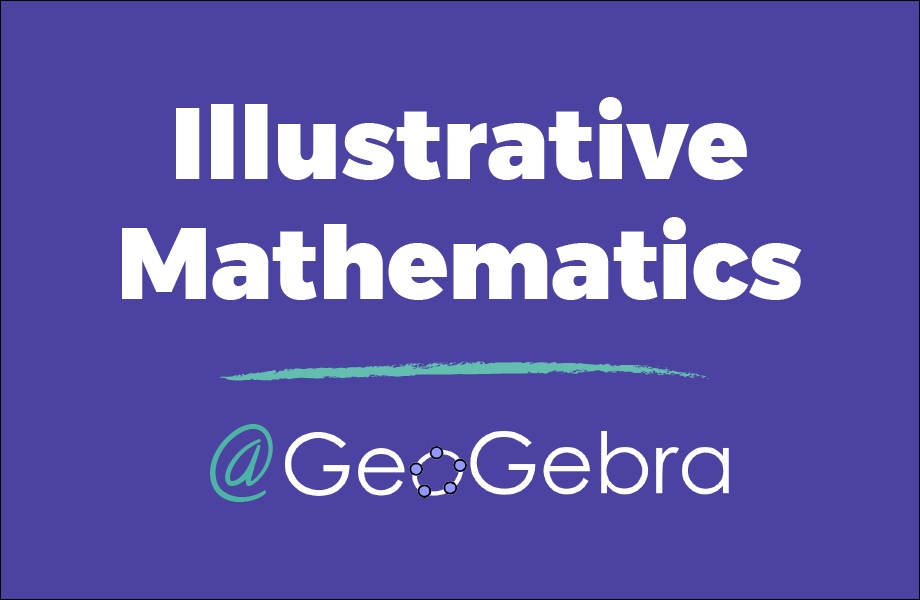### Illustrative Math (6-8) Curriculum

Activity

GeoGebra Classroom Activities

•### solving linear equations

Book

Verna Ashcraft

•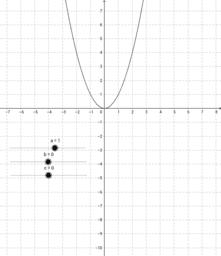Book

Grange

•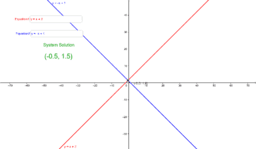### Systems of Equations and Inequalities

Book

dave.dresch

•### Solving Linear Equations

Book

Monica Woo

•### Systems of Equations and Inequalities

Book

ddresch

•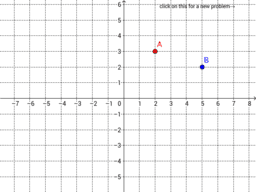### Algebra 1 (Graphs and Equations)

Book

taicahsu

•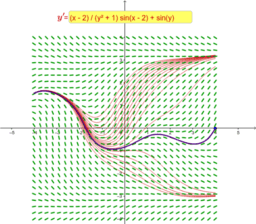### Differential equations

Book

יואב דביר

•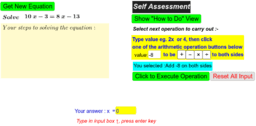### Solving Linear Equations

Book

seacat

•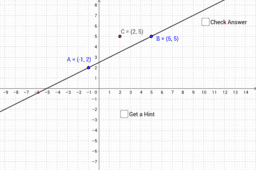### Equations of a straight line

Book

Mr Elliott

•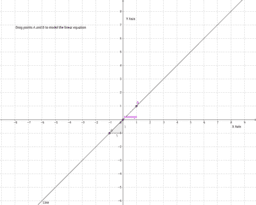### Visuals for Equations

Book

harpjen

•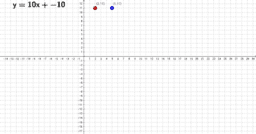### Algebra Linear equations

Book

Kgibson

•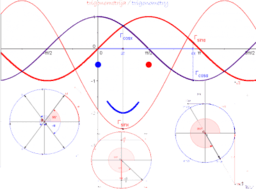### Trigonometrija / Trigonometry

Book

Vesna Babović

•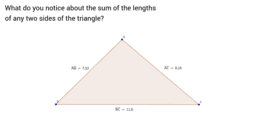### Triangle Inequalities

Book

evazus

•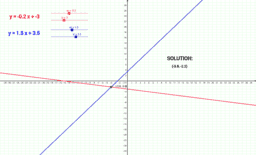### Systems of Equations

Book

cvanloon

•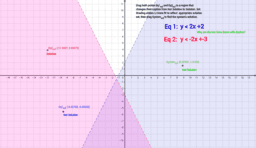### algebra linear equations

Book

•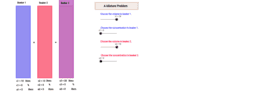### System of Equations

Book

Mr.Healey

•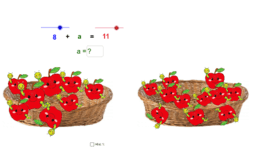### Variables, Equations

Book

Anita83

•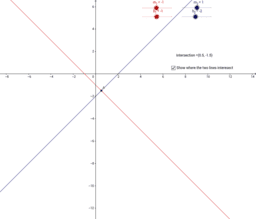### Solving Equations

Book

SteveAMG

•### Coordinate Geometry

Book

Simona Riva

•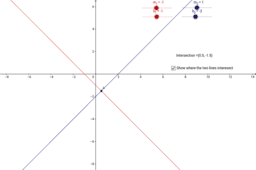### Systems of Linear Equations

Book

hnees

•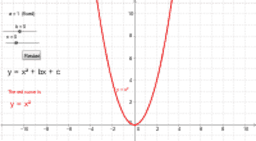Book

Mr Creamer

•### Equations of a Straight Line

Book

AnthElliott

•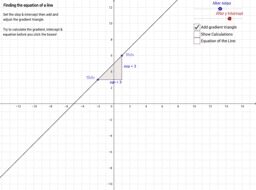Book

DavidA